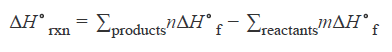Problem: Calculate the standard enthalpy of the reaction, ΔH°rxn, for the thermite reaction:2Al(s) + Fe2O3(s) → 2Fe(s) + Al2O3(s)Elements in their standard state have an enthalpy of formation value of zero. The standard enthalpies of formation of Fe2O3and Al2O3areΔH ∘f of Fe2O3(s) = − 825.5 kJ/ molΔH ∘f of Al2O3(s) = − 1675 kJ/ molExpress your answer to three significant figures and include the appropriate units.Enthalpy (H) is the heat content of a system. The heat change, or enthalpy change, is represented as ΔH.The enthalpy change for a chemical reaction can be given asenthalpy change for the reaction = (enthalpy of formation of the products) − (enthalpy of formation of the reactants)The symbol for the standard enthalpy of reaction is ΔH°rxn, where the subscript "rxn" stands for "reaction." The standard enthalpy of a reaction is calculated from the standard enthalpy of formation values for the reactants and products.The standard enthalpy of formation is denoted as ΔH°f, where the subscript "f" stands for "formation." It is the enthalpy change for the formation of 1 mol of a substance in its standard state from its constituent elements in their standard states. If the standard enthalpies of formation of the reactants and of the products are known, the standard enthalpy of reaction can be calculated using the formulawhere Σ means sum and n and m denote the appropriate stoichiometric coefficients for each substance.

FREE Expert Solution
82% (462 ratings)Problem Details

Calculate the standard enthalpy of the reaction, ΔH°rxn, for the thermite reaction:

2Al(s) + Fe2O3(s) → 2Fe(s) + Al2O3(s)

Elements in their standard state have an enthalpy of formation value of zero. The standard enthalpies of formation of Fe2O3and Al2O3are
ΔH ∘f of Fe2O3(s) = − 825.5 kJ/ mol
ΔH ∘f of Al2O3(s) = − 1675 kJ/ mol

Express your answer to three significant figures and include the appropriate units.

Enthalpy (H) is the heat content of a system. The heat change, or enthalpy change, is represented as ΔH.

The enthalpy change for a chemical reaction can be given as

enthalpy change for the reaction = (enthalpy of formation of the products) − (enthalpy of formation of the reactants)

The symbol for the standard enthalpy of reaction is ΔH°rxn, where the subscript "rxn" stands for "reaction." The standard enthalpy of a reaction is calculated from the standard enthalpy of formation values for the reactants and products.

The standard enthalpy of formation is denoted as ΔH°f, where the subscript "f" stands for "formation." It is the enthalpy change for the formation of 1 mol of a substance in its standard state from its constituent elements in their standard states. If the standard enthalpies of formation of the reactants and of the products are known, the standard enthalpy of reaction can be calculated using the formulawhere Σ means sum and n and m denote the appropriate stoichiometric coefficients for each substance.